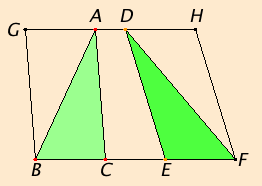# Proposition 38

Triangles which are on equal bases and in the same parallels equal one another.

Let ABC and DEF be triangles on equal bases BC and EF and in the same parallels BF and AD.I say that the triangle ABC equals the triangle DEF.

Produce AD in both directions to G and H. Draw BG through B parallel to CA, and draw FH through F parallel to DE.

I.36

Then each of the figures GBCA and DEFH is a parallelogram, and GBCA equals DEFH, for they are on equal bases BC and EF and in the same parallels BF and GH.

I.34

Moreover the triangle ABC is half of the parallelogram GBCA, for the diameter AB bisects it. And the triangle FED is half of the parallelogram DEFH, for the diameter DF bisects it.

C.N.

Therefore the triangle ABC equals the triangle DEF.

Therefore triangles which are on equal bases and in the same parallels equal one another.

Q.E.D.

## Guide

The idea of the argument is clear: since parallelograms on equal bases and in the same parallels are equal by I.36, and the triangles are half the parallelograms by I.34, therefore the triangles are also equal.

#### Use of Proposition 38

This proposition is used in I.40, I.42, and VI.1.